Sphere A solid having the shape of a ball is called a sphere. [Figure 1-28] A sphere has a constant diameter. The radius (r) of a sphere is one-half of the diameter (D). The formula for the volume of a sphere is given as: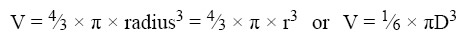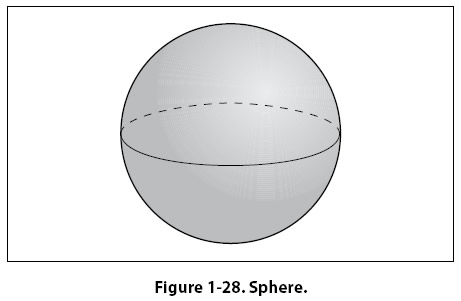Example: A pressure tank inside the fuselage of a cargo aircraft is in the shape of a sphere with a diameter of 34 inches. What is the volume of the pressure tank?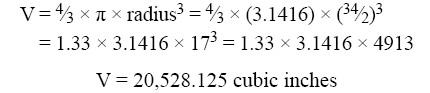Cone A solid with a circle as a base and with sides that gradually taper to a point is called a cone. [Figure 1-29] The formula for the volume of a cone is given as: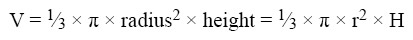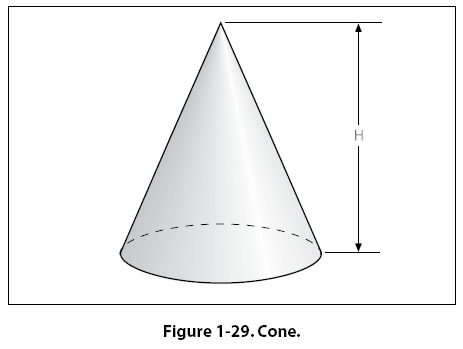Units of Volume Since all volumes are not measured in the same units, it is necessary to know all the common units of volume and how they are related to each other. For example, the mechanic may know the volume of a tank in cubic feet or cubic inches, but when the tank is full of gasoline, he or she will be interested in how many gallons it contains. Refer to Figure 1-37, Applied Mathematics Formula Sheet, at the end of the chapter for a comparison of different units of volume.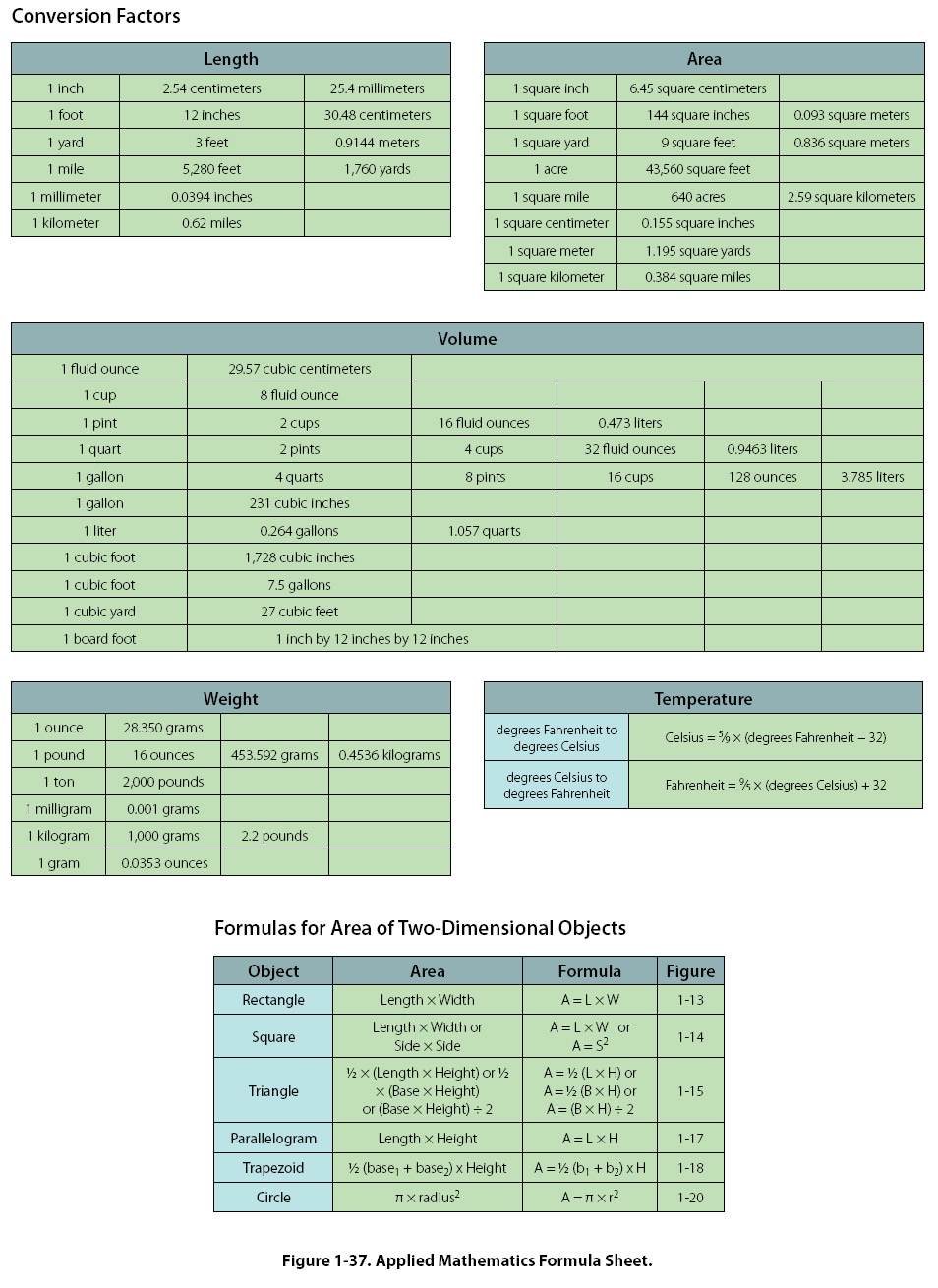Computing Surface Area of Three-dimensional Solids The surface area of a three-dimensional solid is the sum of the areas of the faces of the solid. Surface area is a different concept from that of volume. For example, surface area is the amount of sheet metal needed to build a rectangular fuel tank while volume is the amount of fuel that the tank can contain. Rectangular Solid The formula for the surface area of a rectangular solid [Figure 1-24] is given as: Surface Area = 2 x [(Width x Length) + (Width x Height) + (Length x Height)] = 2 x [(W x L) + (W x H) + (L x H)]
 ©AvStop Online Magazine                                                                                                                                                      Contact Us              Return To Books